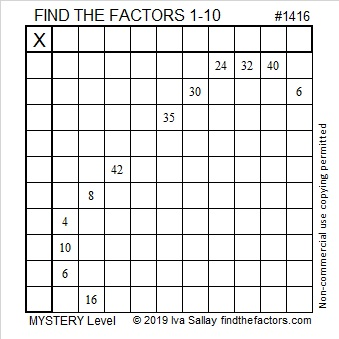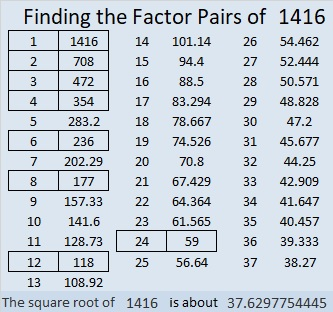1416 A Birthday Mystery

Today is my sister’s birthday, but the cake is tipped over and there’s a big hole in it! And what happened to the candle? Can you solve this mystery? Happy birthday, Sue!Print the puzzles or type the solution in this excel file: 10 Factors 1410-1418

Now I’ll share some facts about the puzzle number 1416:

• 1416 is a composite number.
• Prime factorization: 1416 = 2 × 2 × 2 × 3 × 59, which can be written 1416 = 2³ × 3 × 59
• 1416 has at least one exponent greater than 1 in its prime factorization so √1416 can be simplified. Taking the factor pair from the factor pair table below with the largest square number factor, we get √1416 = (√4)(√354) = 2√354
• The exponents in the prime factorization are 3,1 and 1. Adding one to each exponent and multiplying we get (3 + 1)(1 + 1) (1 + 1) = 4 × 2 × 2 = 16. Therefore 1416 has exactly 16 factors.
• The factors of 1416 are outlined with their factor pair partners in the graphic below.1416 is the difference of two squares four ways:
355² – 353²  = 1416
179² – 175²  = 1416
121² – 115²  = 1416
65² – 53²  = 1416